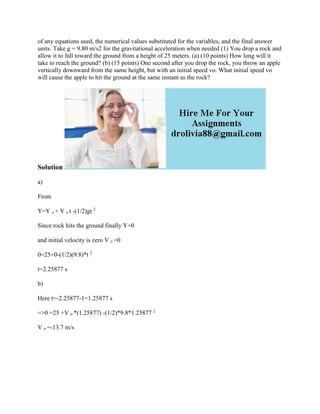Diese Präsentation wurde erfolgreich gemeldet.

# of any equations used- the numerical values substituted for the variab.docx

Anzeige
Anzeige
Anzeige
Anzeige
Anzeige
Anzeige
Anzeige
Anzeige
Anzeige
Anzeige
Anzeige×

1 von 2 Anzeige

# of any equations used- the numerical values substituted for the variab.docx

of any equations used, the numerical values substituted for the variables, and the final answer units. Take g = 9.80 m/s2 for the gravitational acceleration when needed (1) You drop a rock and allow it to fall toward the ground from a height of 25 meters. (a) (10 points) How long will it take to reach the ground? (b) (15 points) One second after you drop the rock, you throw an apple vertically downward from the same height, but with an initial speed vo. What initial speed vo will cause the apple to hit the ground at the same instant as the rock?
Solution
a)
From
Y=Y o + V o t -(1/2)gt 2
Since rock hits the ground finally Y=0
and initial velocity is zero V o =0
0=25+0-(1/2)(9.8)*t 2
t=2.25877 s
b)
Here t=-2.25877-1=1.25877 s
=>0 =25 +V o *(1.25877) -(1/2)*9.8*1.25877 2
V o =-13.7 m/s
.

of any equations used, the numerical values substituted for the variables, and the final answer units. Take g = 9.80 m/s2 for the gravitational acceleration when needed (1) You drop a rock and allow it to fall toward the ground from a height of 25 meters. (a) (10 points) How long will it take to reach the ground? (b) (15 points) One second after you drop the rock, you throw an apple vertically downward from the same height, but with an initial speed vo. What initial speed vo will cause the apple to hit the ground at the same instant as the rock?
Solution
a)
From
Y=Y o + V o t -(1/2)gt 2
Since rock hits the ground finally Y=0
and initial velocity is zero V o =0
0=25+0-(1/2)(9.8)*t 2
t=2.25877 s
b)
Here t=-2.25877-1=1.25877 s
=>0 =25 +V o *(1.25877) -(1/2)*9.8*1.25877 2
V o =-13.7 m/s
.

Anzeige
Anzeige

### of any equations used- the numerical values substituted for the variab.docx

1. 1. of any equations used, the numerical values substituted for the variables, and the final answer units. Take g = 9.80 m/s2 for the gravitational acceleration when needed (1) You drop a rock and allow it to fall toward the ground from a height of 25 meters. (a) (10 points) How long will it take to reach the ground? (b) (15 points) One second after you drop the rock, you throw an apple vertically downward from the same height, but with an initial speed vo. What initial speed vo will cause the apple to hit the ground at the same instant as the rock? Solution a) From Y=Y o + V o t -(1/2)gt 2 Since rock hits the ground finally Y=0 and initial velocity is zero V o =0 0=25+0-(1/2)(9.8)*t 2 t=2.25877 s b) Here t=-2.25877-1=1.25877 s =>0 =25 +V o *(1.25877) -(1/2)*9.8*1.25877 2 V o =-13.7 m/s